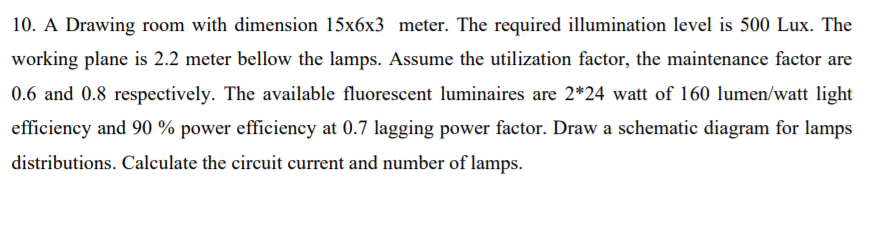# 10. A Drawing room with dimension 15x6x3 meter. The required illumination level is 500 Lux. The working plane is 2.2 meter bellow the lamps. Assume the utilization factor, the maintenance factor are 0.6 and 0.8 respectively. The available fluorescent luminaires are 2*24 watt of 160 lumen/watt light efficiency and 90 % power efficiency at 0.7 lagging power factor. Draw a schematic diagram for lamps distributions. Calculate the circuit current and number of lamps.

Questionhelp_outlineImage Transcriptionclose10. A Drawing room with dimension 15x6x3 meter. The required illumination level is 500 Lux. The working plane is 2.2 meter bellow the lamps. Assume the utilization factor, the maintenance factor are 0.6 and 0.8 respectively. The available fluorescent luminaires are 2*24 watt of 160 lumen/watt light efficiency and 90 % power efficiency at 0.7 lagging power factor. Draw a schematic diagram for lamps distributions. Calculate the circuit current and number of lamps. fullscreen

### Want to see this answer and more?

Experts are waiting 24/7 to provide step-by-step solutions in as fast as 30 minutes!*

*Response times may vary by subject and question complexity. Median response time is 34 minutes for paid subscribers and may be longer for promotional offers.
Tagged in
Engineering
Electrical Engineering

### Other